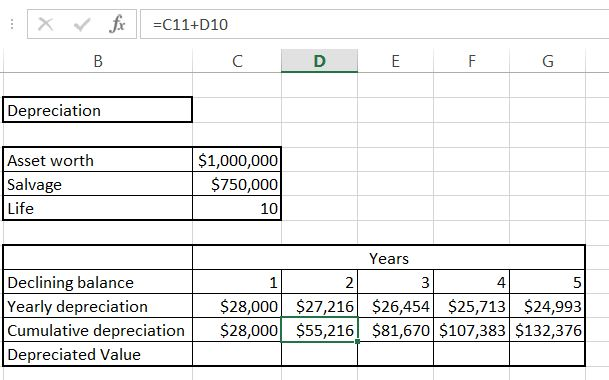## Declining Balance

In this Excel lesson you will learn yourself how to calculate Declining Balance Depreciation.
We are having data about an asset in your balance sheet.The asset:

• it is 1 million of dollars worth
• salvage value is \$750 000
• life of the asset is 10 yearsTo calculate Declining Balance we will use DB Excel formula.

Syntax of Declining Balance Excel formula is:

• cost
• salvage
• life
• period

Luckily we already have all data we need.

To calculate yearly depreciation use =DB(\$C\$4,\$C\$5,\$C\$6,C9) formula.Absolute reference is needed because the data will not change in the table. Only the last arguments changes because it is the value of the year.

Cumulative depreciation is just =C11+D10. Drag it right to calculate all of them. This is just current year value plus sum of previous ones.Deprecated value is =\$C\$4-C11 which is the starting value reduced by cumulative depreciation.This is Declining Balance formula and the easiest way to calculate Declining Balance Depreciation in Excel.

The difference between straight line depreciation and declining balance is that for straight line depreciation yearly depreciation is the same value and for declining balance it is different (based on percentage).

## Template

```Further reading: